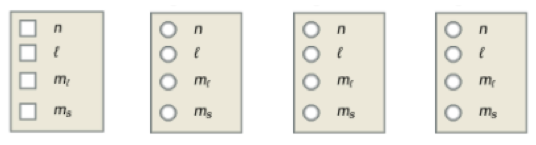# Problem: Answer each of the following questions.a) What information is needed to determine the energy of an electron in a many-electron atom? Check all that apply.b) What information is most important in determining the size of an orbital? c) What information is needed to determine the orientation of an orbital? d) What information is needed to determine the general shape of an orbital?

###### FREE Expert Solution
89% (156 ratings)
###### FREE Expert Solution

a) Principle quantum number $n$, which determines the size and energy, is needed to determine the energy of an electron in a many-electron atom.

b) Principle quantum number $n$ is needed to determine the size of an orbital.

89% (156 ratings)###### Problem Details

Answer each of the following questions.

a) What information is needed to determine the energy of an electron in a many-electron atom? Check all that apply.

b) What information is most important in determining the size of an orbital?

c) What information is needed to determine the orientation of an orbital?

d) What information is needed to determine the general shape of an orbital?Frequently Asked Questions

What scientific concept do you need to know in order to solve this problem?

Our tutors have indicated that to solve this problem you will need to apply the Introduction to Quantum Mechanics concept. You can view video lessons to learn Introduction to Quantum Mechanics. Or if you need more Introduction to Quantum Mechanics practice, you can also practice Introduction to Quantum Mechanics practice problems.

What professor is this problem relevant for?

Based on our data, we think this problem is relevant for Professor Young's class at NOTRE DAME.